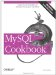# Summarizing with SUM( ) and AVG( )

### Summarizing with SUM() and AVG()

7.4.1 Problem

You need to add up a set of numbers or find their average.

7.4.2 Solution

Use the SUM( ) or AVG( ) functions.

7.4.3 Discussion

SUM( ) and AVG( ) produce the total and average (mean) of a set of values:

• What is the total amount of mail traffic and the average size of each message?

```mysql> SELECT SUM(size) AS 'total traffic',
-> AVG(size) AS 'average message size'
-> FROM mail;
+---------------+----------------------+
| total traffic | average message size |
+---------------+----------------------+
| 3798185 | 237386.5625 |
+---------------+----------------------+```
• How many miles did the drivers in the driver_log table travel? What was the average miles traveled per day?

```mysql> SELECT SUM(miles) AS 'total miles',
-> AVG(miles) AS 'average miles/day'
-> FROM driver_log;
+-------------+-------------------+
| total miles | average miles/day |
+-------------+-------------------+
| 2166 | 216.6000 |
+-------------+-------------------+```
• What is the total population of the United States?

```mysql> SELECT SUM(pop) FROM states;
+-----------+
| SUM(pop) |
+-----------+
| 248102973 |
+-----------+```

(The value represents the population reported for April, 1990. The figure shown here differs from the U.S. population reported by the U.S. Census Bureau, because the states table doesn't contain a count for Washington, D.C.)

SUM( ) and AVG( ) are strictly numeric functions, so they can't be used with strings or temporal values. On the other hand, sometimes you can convert non-numeric values to useful numeric forms. Suppose a table stores TIME values that represent elapsed time:

```mysql> SELECT t1 FROM time_val;
+----------+
| t1 |
+----------+
| 15:00:00 |
| 05:01:30 |
| 12:30:20 |
+----------+```

To compute the total elapsed time, use TIME_TO_SEC( ) to convert the values to seconds before summing them. The result also will be in seconds; pass it to SEC_TO_TIME( ) should you wish the sum to be in TIME format:

```mysql> SELECT SUM(TIME_TO_SEC(t1)) AS 'total seconds',
-> SEC_TO_TIME(SUM(TIME_TO_SEC(t1))) AS 'total time'
-> FROM time_val;
+---------------+------------+
| total seconds | total time |
+---------------+------------+
| 117110 | 32:31:50 |
+---------------+------------+```

7.4.4 See Also

The SUM( ) and AVG( ) functions are especially useful in applications that compute statistics. They're explored further in Chapter 13, along with STD( ), a related function that calculates standard deviations.MySQL Cookbook
ISBN: 059652708X
EAN: 2147483647
Year: 2005
Pages: 412
Authors: Paul DuBois

Similar book on Amazon

Flylib.com © 2008-2020.
If you may any questions please contact us: flylib@qtcs.net